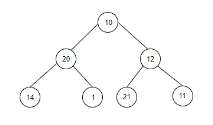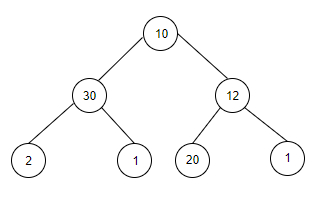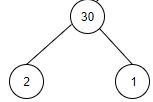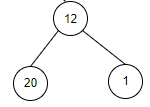# Count subtrees that sum up to a given value x in C++

Given a binary tree and a value x as input. The goal is to find all the subtrees of a binary tree that have sum of weights of its nodes equal to x.

For Example

## Input

x = 14. The tree which will be created after inputting the values is given below## Output

Count of subtrees that sum up to a given value x are: 1

## Explanation

we are given with a x value as 14. As we can see there is only one leaf node with the values as 14 therefore the count is 1.

## Input

x = 33. The tree which will be created after inputting the values is given below −## Output

Count of subtrees that sum up to a given value x are: 2

## Explanation

we are given with a x value as 33. As we can see there are two subtrees
with the sum values as 33 therefore the count is 2.Approach used in the below program is as follows

In this approach we will recursively calculate the sum of weights of the root node’s left subtree and right subtree and in the end we will add it to the root's weight. If sum is equal to x then increment count.

• Construct a tree Tree_Node with root as a pointer to its root.

• Function insert_Node(int data) adds nodes to this tree.

• Function subtrees_x(Tree_Node* root, int x) takes the root pointer to the tree and x and returns the count of subtrees that sum up to a given value x.

• Take a static variable count as 0 as we will calculate count recursively.

• Take a static node of type Tree_node as root.

• Initialize variables Left_subtree = 0, Right_subtree = 0. For sum of node’s weights in left and right subtrees from the root.

• If root is NULL, return sum as 0.

• Calculate Left_subtree += subtrees_x(root−>Left, x) for sum of nodes in left subtree.

• Calculate Right_subtree += subtrees_x(root−>Right, x) for sum of nodes in left subtree.

• Set sum=Left_subtree + Right_subtree + root−>ldata.

• If sum is equal to x then increment count.

• If temp!=root, not the start node, then return sum as Left_subtree + root−>data + Right_subtree.

• At the end return count as desired count of trees with node’s sum equal to x.

## Example

Live Demo

#include <bits/stdc++.h>
using namespace std;
struct Tree_Node{
int data;
Tree_Node *Left, *Right;
};
Tree_Node* insert_Node(int data){
Tree_Node* new_node = (Tree_Node*)malloc(sizeof(Tree_Node));
new_node−>data = data;
new_node−>Left = new_node−>Right = NULL;
return new_node;
}
int subtrees_x(Tree_Node* root, int x){
static int count = 0;
static Tree_Node* temp = root;
int Left_subtree = 0, Right_subtree = 0;
if(root == NULL){
return 0;
}
Left_subtree += subtrees_x(root−>Left, x);
Right_subtree += subtrees_x(root−>Right, x);
int sum = Left_subtree + Right_subtree + root−>data;
if(sum == x){
count++;
}
if(temp != root){
int set = Left_subtree + root−>data + Right_subtree;
return set;
}
return count;
}
int main(){
Tree_Node* root = insert_Node(10);
root−>Left = insert_Node(20);
root−>Right = insert_Node(12);
root−>Left−>Left = insert_Node(14);
root−>Left−>Right = insert_Node(1);
root−>Right−>Left = insert_Node(21);
root−>Right−>Right = insert_Node(11);
int x = 14;
cout<<"Count of subtrees that sum up to a given value x are: "<<subtrees_x(root, x);
return 0;
}

## Output

If we run the above code it will generate the following output −

Count of subtrees that sum up to a given value x are: 1